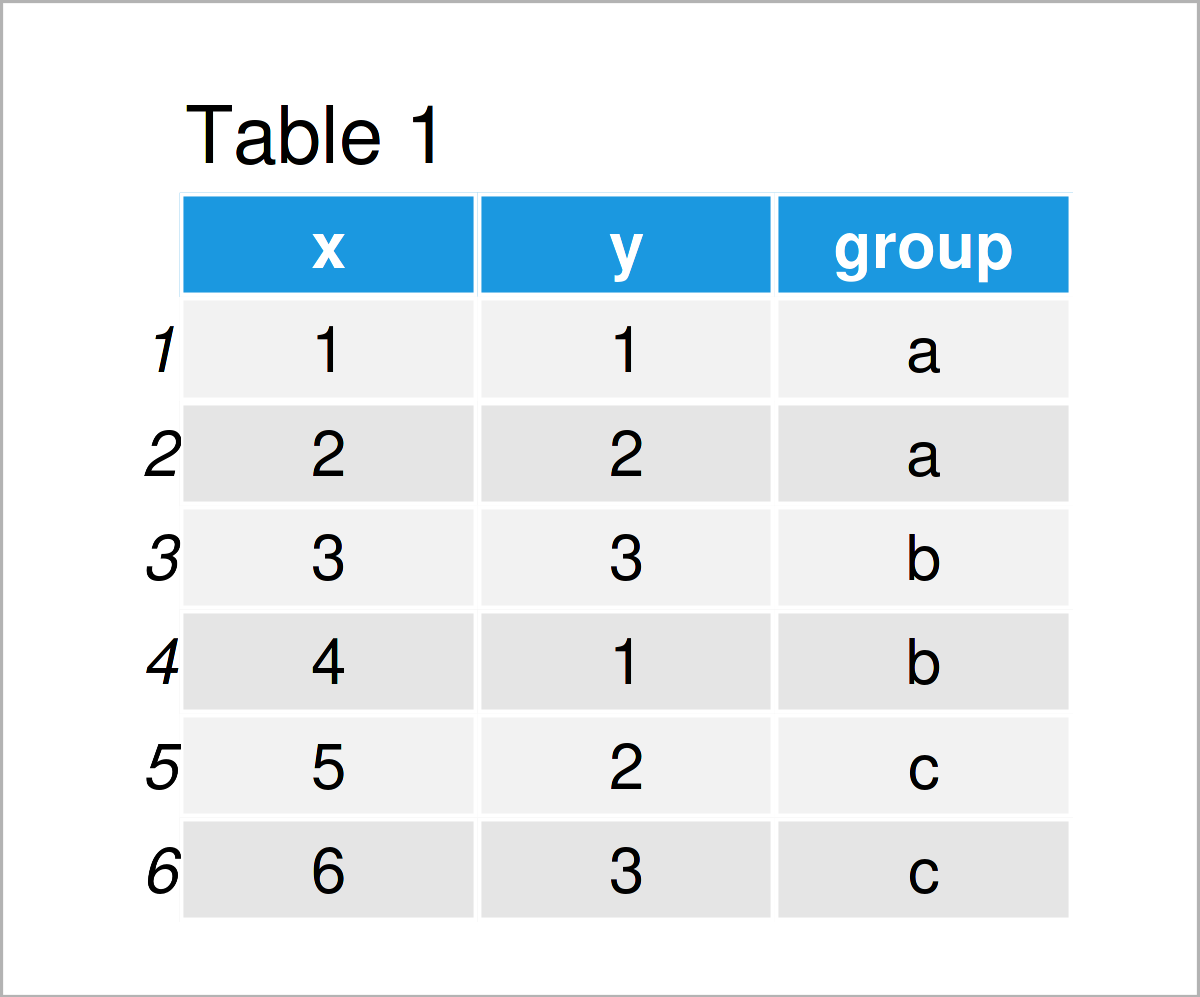# R ggplot2 geom_path Error in FUN(X[[i]], …) object not found (2 Examples)

In this tutorial, I’ll explain how to reproduce and debug the “Error in FUN(X[[i]], …) : object not found” when using the geom_path function of the ggplot2 package in R programming.

The tutorial will consist of the following contents:

Here’s how to do it:

## Example Data, Add-On Packages & Default Plot

The following data will be used as a basis for this R programming tutorial:

```data <- data.frame(x = 1:6,                     # Create example data frame
y = 1:3,
group = rep(letters[1:3], each = 2))
data                                            # Print example data frame```Table 1 visualizes the structure of our example data: It is composed of six rows and three columns.

We also need to install and load the ggplot2 package, to be able to use the corresponding functions.

```install.packages("ggplot2")                     # Install & load ggplot2 package
library("ggplot2")```

Now, we can draw a ggplot2 plot of our data as follows:

```ggp <- ggplot(data,                             # Create ggplot2 plot without path
aes(x = x,
y = y,
col = group)) +
geom_point()
ggp                                             # Draw ggplot2 plot without path```By executing the previous R programming syntax, we have plotted Figure 1, i.e. a ggplot2 scatterplot without a path.

Let’s specify the x- and y-axis coordinates for our path:

```data_path <- data.frame(x_path = c(1, 3, 5),    # Specify data for path
y_path = c(3, 2, 3))
data_path                                       # Print data for path```As shown in Table 2, the previous R code has created a new data set called data_path, which contains all the coordinates of our path.

Next, we would like to add this path on top of our plot using the geom_path function. However, this can lead to problems…

## Example 1: Reproduce the Error in FUN(X[[i]], …) : object ‘group’ not found

In this example, I’ll show how to replicate the “Error in FUN(X[[i]], …) : object not found”.

Let’s assume that we want to add a path to our plot using the geom_path function. Then, we might try to do this as shown below:

```ggp +                                           # Trying to draw path
geom_path(data = data_path,
aes(x = x_path,
y = y_path))

As you can see, the previous R code has returned the error message “Error in FUN(X[[i]], …) : object ‘group’ not found”. This is because the geom_path function searches for the group variable in our path data set.

Let’s solve this issue!

## Example 2: Fix the Error in FUN(X[[i]], …) : object ‘group’ not found

In this example, I’ll show how to debug the “Error in FUN(X[[i]], …) : object not found”.

To accomplish this, we have to set the inherit.aes argument within the geom_path function to FALSE.

Consider the R syntax below:

```ggp +                                           # Drawing path properly
geom_path(data = data_path,
aes(x = x_path,
y = y_path),
inherit.aes = FALSE)```As shown in Figure 2, we have plotted a ggplot2 scatterplot with a path on top. No error messages are shown anymore.

## Video & Further Resources

Have a look at the following video which I have published on my YouTube channel. In this tutorial, I give a detailed introduction to the ggplot2 Package and data visualization in R, structured in different sections with examples for beginners but also advanced users.

Please accept YouTube cookies to play this video. By accepting you will be accessing content from YouTube, a service provided by an external third party.If you accept this notice, your choice will be saved and the page will refresh.

Furthermore, you may have a look at some of the other posts on this website. Please find a selection of posts below.

Subscribe to the Statistics Globe Newsletter

•trilisser
March 29, 2023 8:26 am

Thank you so much! That sh*t has really pissed me off.

•March 31, 2023 8:25 am